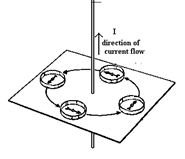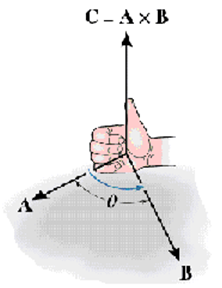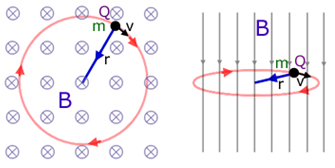# Topic: Moving Charges and Magnetism (Test 1)

Topic: Moving Charges and Magnetism
Q.1
Lorentz Force generally refers to
A. force experienced by a charge due to combined action of electrical fields
B. force experienced by a charge due to combined action of electrical and magnetic fields
C. force experienced by a charge due to action of magnetic fields
D. force experienced by a charge due to combined action of electrical,magnetic field and gravitational field
Answer : Option C
Explaination / Solution:

A moving charge experiences a force in a magnetic field called magnetic Lorentz force given by
Workspace
Report
Q.2
A wire is perpendicular to the plane of the paper. A ring of compass needles surrounds the wire in the plane of the paper with center of ring being the center of the wire .Initially there is no current in the wire. What happens after a steady dc current is established in the wire?
A. The needles become tangential to the ring of which they are a part
B. some needles become parallel to each other while others are uneffected
C. nothing happens
D. The needles become parallel to each other
Answer : Option A
Explaination / Solution:

The current carrying wire has a magnetic field around it and the lines of force are in the form of concentric circles with their centers on the wire.Workspace
Report
Q.3
correct unit of magnetic field is
A. ampere turns
B. Amperes
C. Newton coulombs
D. Tesla
Answer : Option D
Explaination / Solution:

The SI unit of magnetic field is Tesla (T)

Workspace
Report
Q.4
Which of the following is most general charachteristic of Magnetic field
A. The magnetic field exerts a force $\stackrel{}{F}$ on any other moving charge or current that is present in the field.
B. The magnetic field exerts a force $\stackrel{}{F}$ only on any iron filings
C. The magnetic field exerts a force $\stackrel{}{F}$ on any other particle
D. The magnetic field exerts a force $\stackrel{}{F}$ only on any magnets present in the field.
Answer : Option A
Explaination / Solution:

A moving charge experiences a force in a magnetic field called magnetic Lorentz force given by.This force acts only if the particle (i) has charge q(ii) has a non zero velocity v and (iii) does not move parallel or anti parallel to the magnetic field B. A current carrying conductor placed in a magnetic field experiences a force given by
Workspace
Report
Q.5
which of the following best describes the difference between electric and magnetic fields
A. The electric force acts on negative charges in the electric field, whereas the magnetic force acts on positive charges in the magnetic field
B. The electric force acts in the direction of the electric field, whereas the magnetic force acts perpendicular to the magnetic field
C. The electric force acts on positive charges in the electric field, whereas the magnetic force acts on negative charges in the magnetic field
D. The electric force acts in the direction perpendicular to electric field, whereas the magnetic force acts parallel to the magnetic field
Answer : Option B
Explaination / Solution:

The electrostatic force experienced by a positive charge q placed in an electric field is . The force acts in the direction of the electric field.The magnetic force acting on a charge of magnitude q moving with a velocity 'v' is .This acts in a direction perpendicular to the plane containing the vectors.$\stackrel{}{v}$ and
Workspace
Report
Q.6
correct expression for force on a current carrying conductor of length dl in a magnetic field is
A. F⃗ =Idl×2B⃗
B. F⃗ =Idl×B⃗
C. F⃗ =ϵ0Idl×B⃗
D. F⃗ =2Idl×B⃗
Answer : Option B
Explaination / Solution:

force experienced by a conductor of length l carrying current I placed in magnetic field B is given by .Here $\stackrel{\to }{l}$=

Workspace
Report
Q.7
direction of magnetic force on a positive charge moving in a magnetic field is given by
A. left hand rule
B. two hand rule
C. right hand rule
D. thumb rule
Answer : Option C
Explaination / Solution:

The force experienced by a charged particle in a magnetic field is given by .The right hand rule for vector product can be used to determine the direction of $\stackrel{}{F}$.Workspace
Report
Q.8
The magnitude of the magnetic field due to a circular coil of radius R carrying a current I at an axial distance x from the centre is
A. B=μ0IR23(x2+R2)32
B. B=μ0IR22(x2+R2)32
C. B=μ0IR32(x2+R2)32
D. B=μ0IR22(x2+R2)35
Answer : Option B
Explaination / Solution:

magnetic field at a point located at a distance x from the center of the coil of radius R carrying current along its axis is
Workspace
Report
Q.9
Consider the motion of a charged particle in a uniform magnetic field directed into the paper.If velocity v of the particle is in the plane of the paper the charged particle will
A. describe a straight line
B. describe a circle
C. describe an ellipse
D. describe a hyperbola
Answer : Option B
Explaination / Solution:

The charged particle experiences magnetic Lorentz force given by.This force acts in the plane of the paper perpendicular to the velocity vector at all points on the plane. Magnetic Lorentz force acts like a centripetal force, causing the particle to move in a circle.Workspace
Report
Q.10
cyclotron is used to
A. decelerate charged particles or ions to high voltages
B. accelerate charged particles or ions to high energies
C. accelerate charged particles or ions to high voltages
D. accelerate charged particles or ions to low voltages
Answer : Option B
Explaination / Solution:

Cyclotron is a particle accelerator, which uses electric field to accelerate the charged particle and magnetic field to make it move in a circular path. During acceleration in the electric field, the particle acquires high energies of the order of a few million electron volts.

Workspace
Report